# Selina Solutions Concise Maths Class 10 Chapter 16 Loci (Locus and its Constructions) Exercise 16(B)

Different kinds of locus and its constructions are practised under this exercise. The Selina Solutions for Class 10 Maths is the best reference one could get for clarifying doubts and to increase problem-solving skills.The solutions are created by subject matter experts, according to the latest ICSE patterns. Further, the solution PDF of the Selina Solutions Concise Maths Class 10 Chapter 16 Loci (Locus and its Constructions) Exercise 16(B) is provided in the link given below.

## Selina Solutions Concise Maths Class 10 Chapter 16 Loci (Locus and its Constructions) Exercise 16(B) Download PDF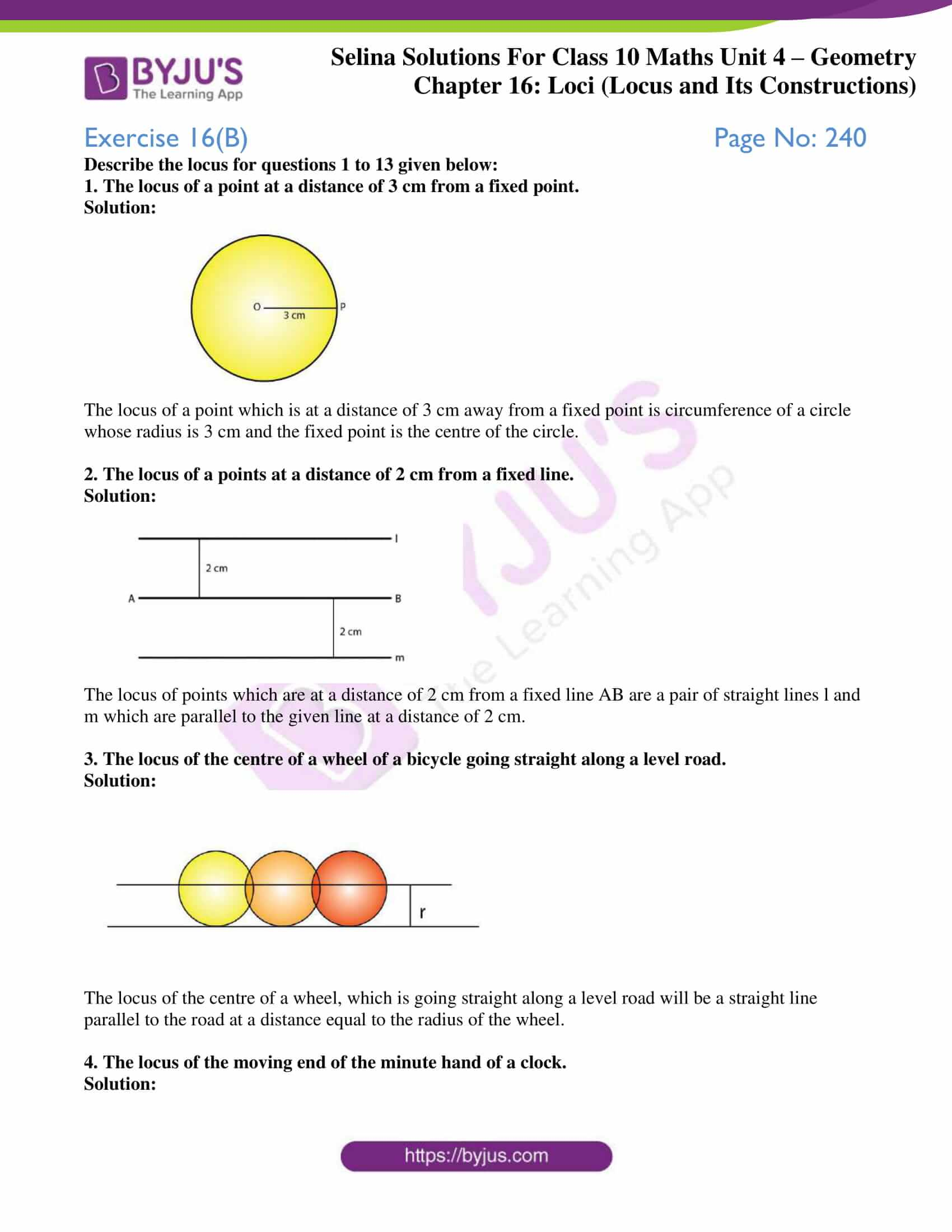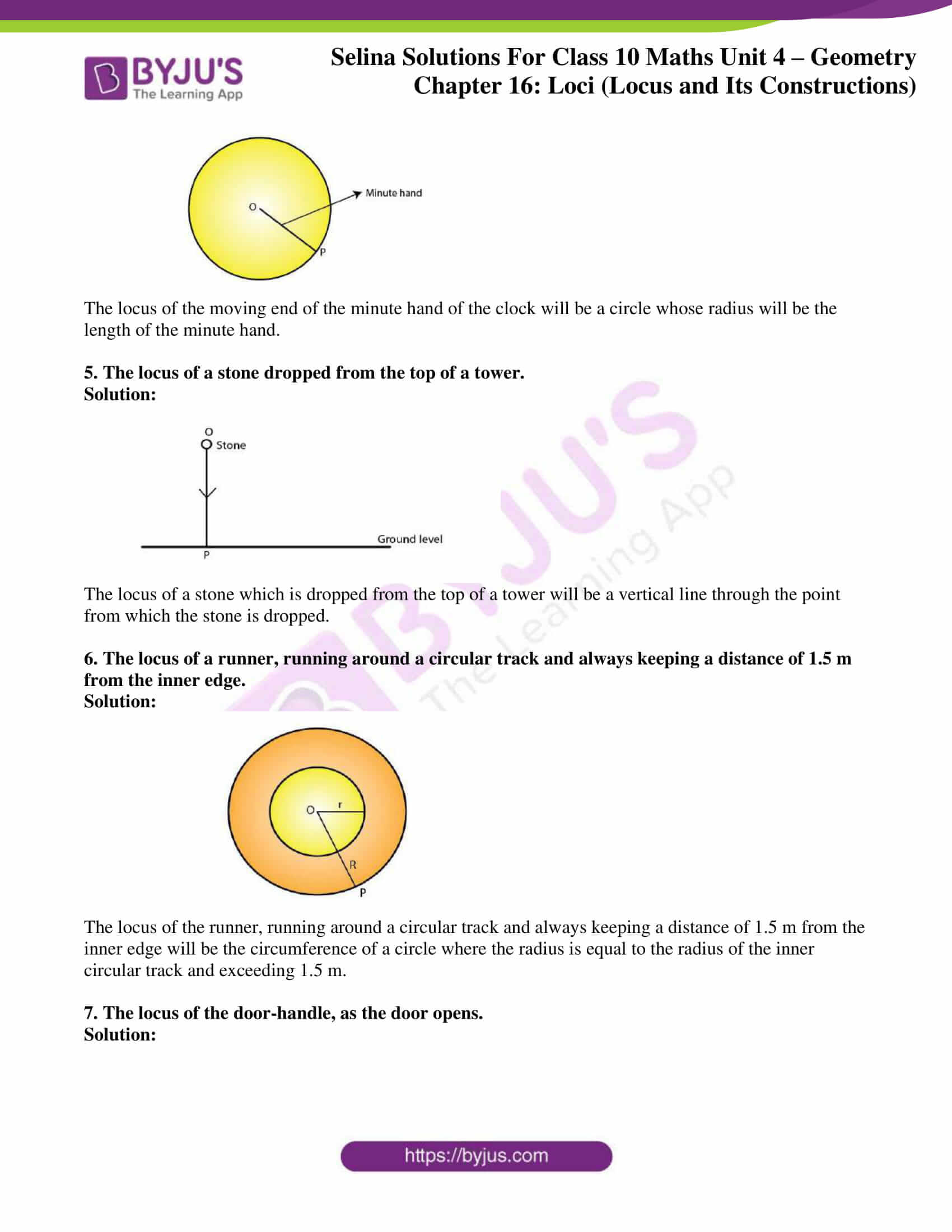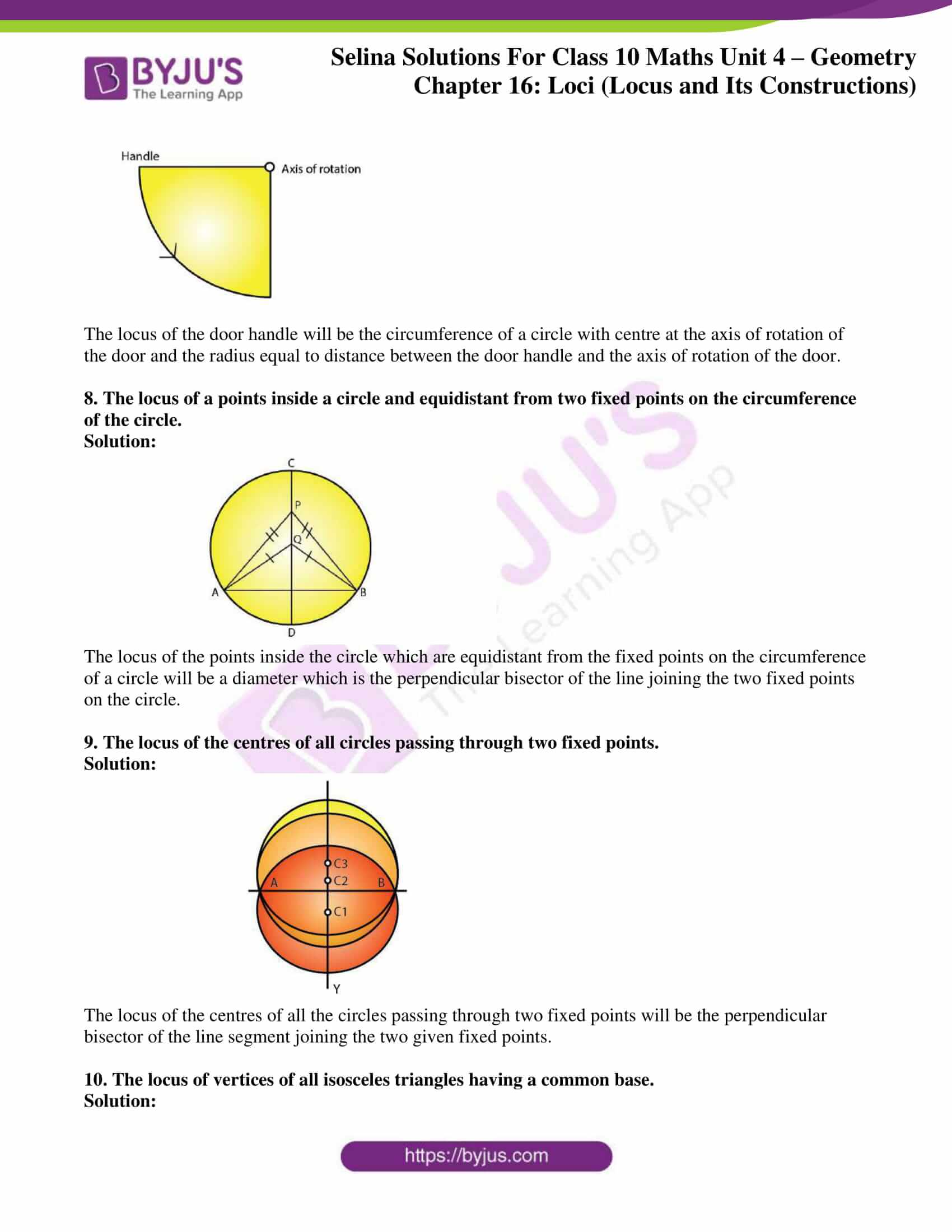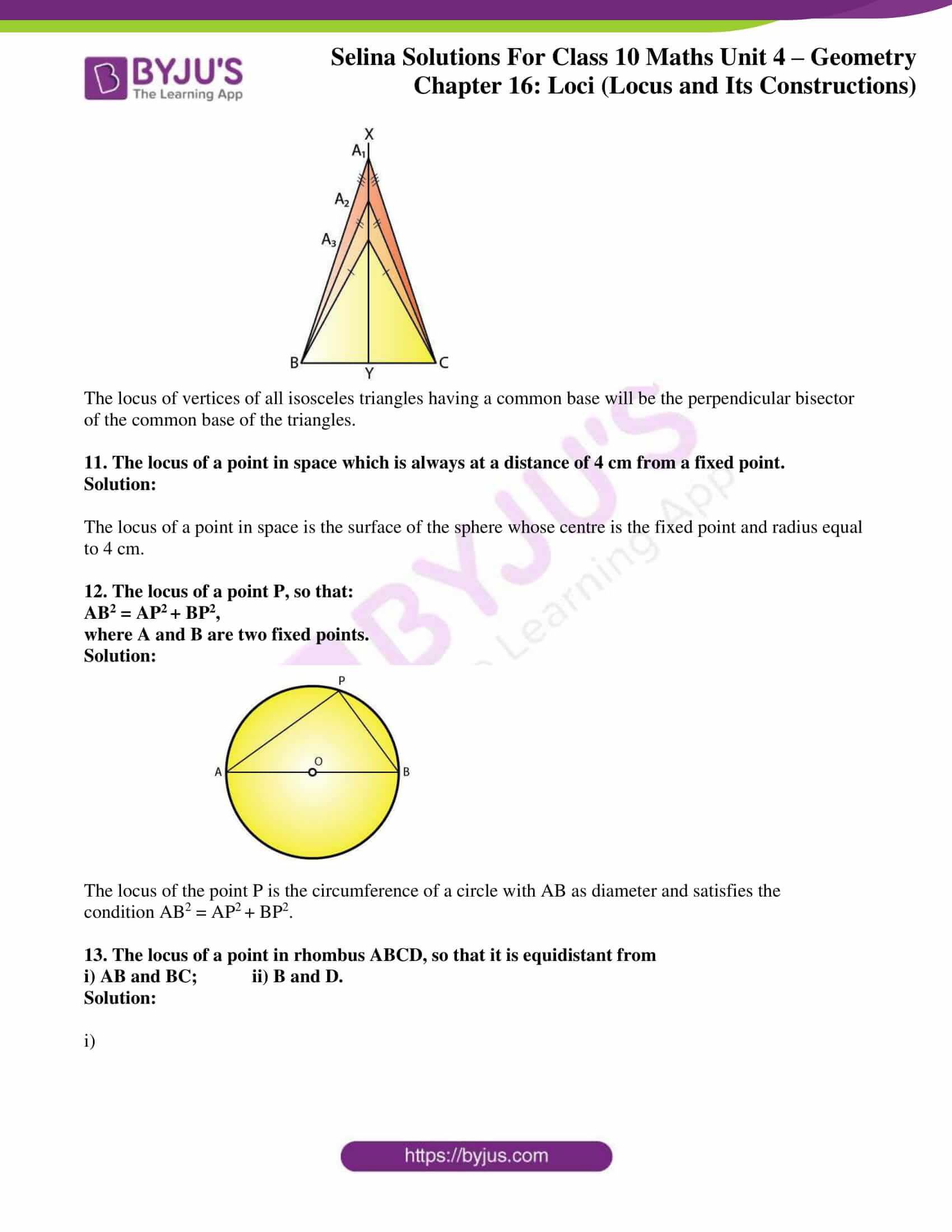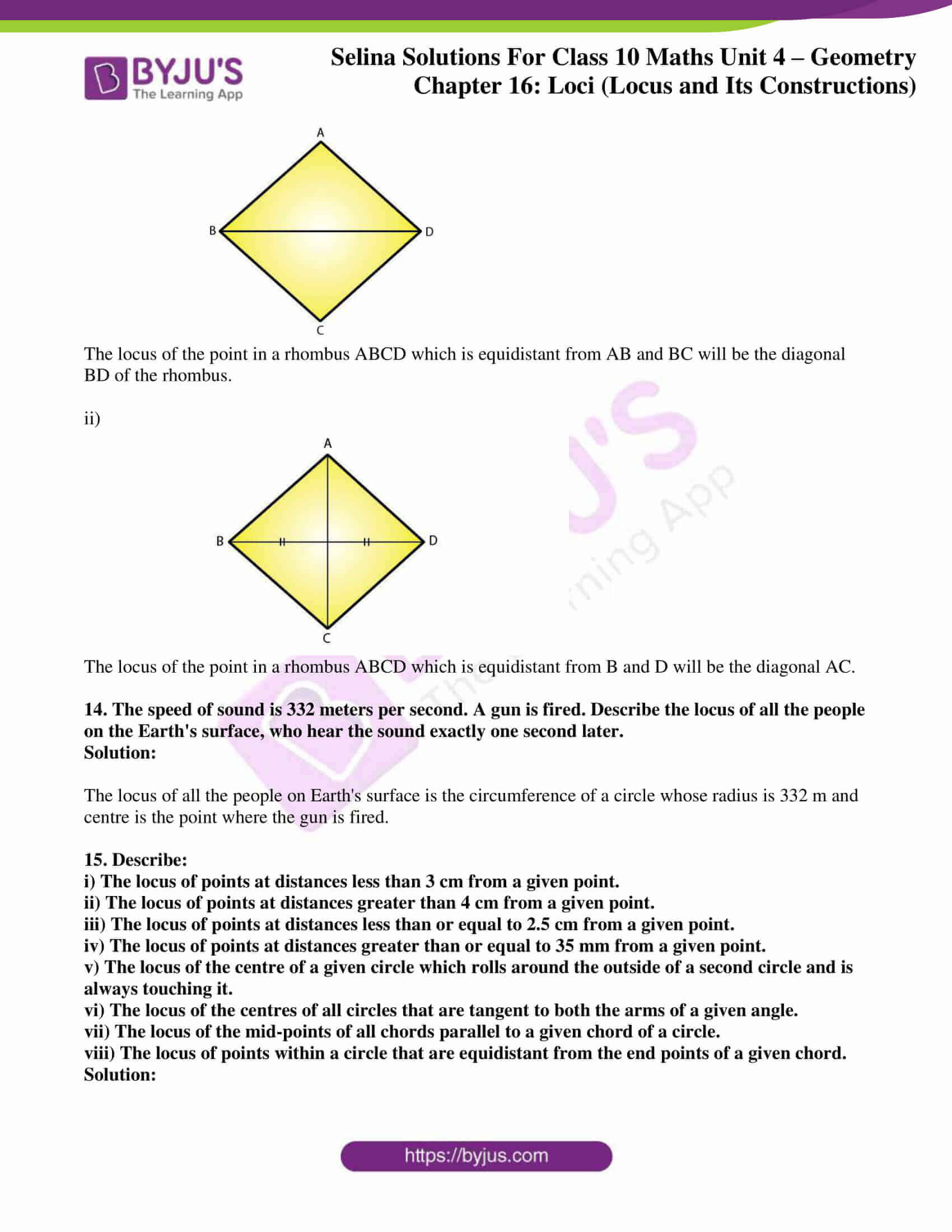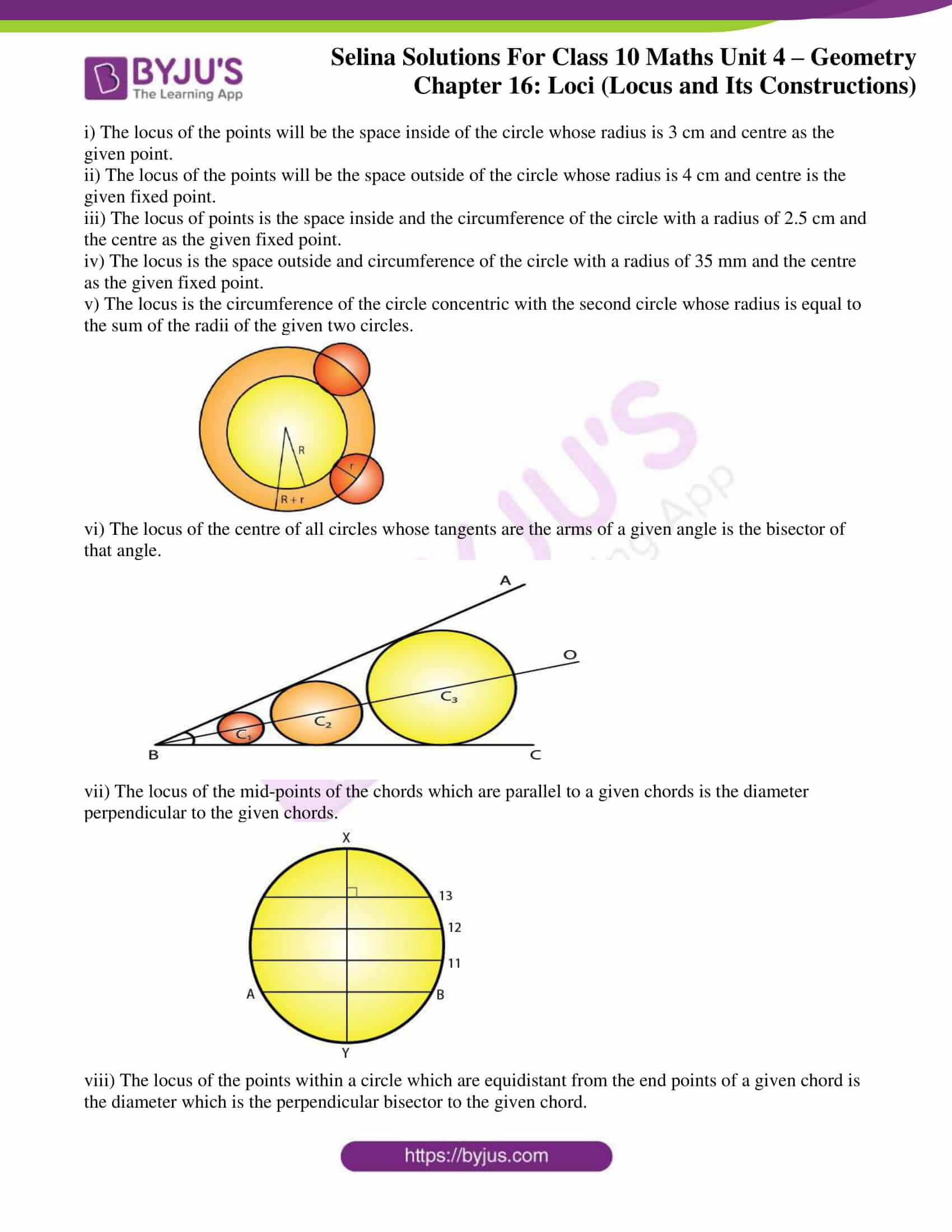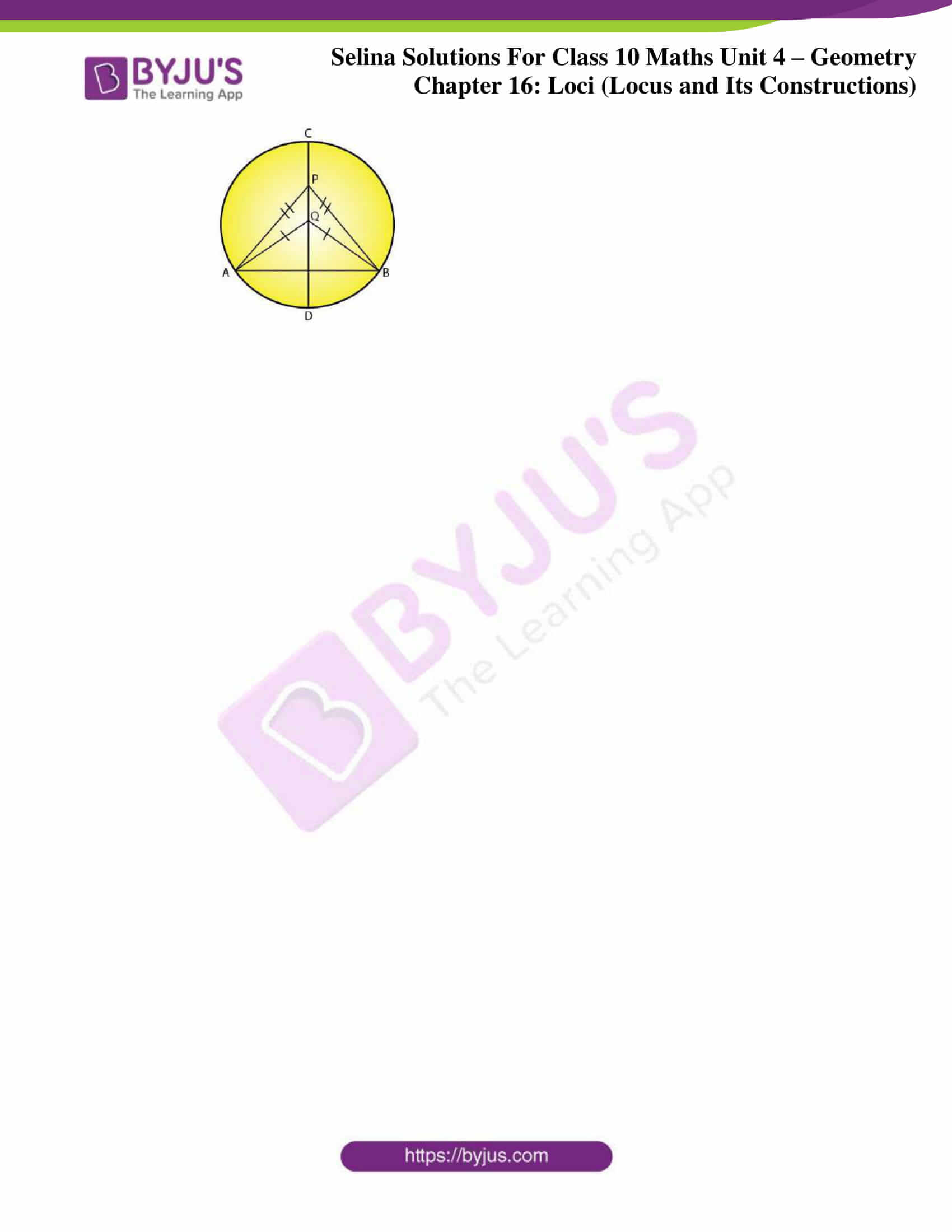### Access Selina Solutions Concise Maths Class 10 Chapter 16 Loci (Locus and its Constructions) Exercise 16(B)

#### Exercise 16(B) Page No: 240

Describe the locus for questions 1 to 13 given below:

1. The locus of a point at a distance of 3 cm from a fixed point.

Solution: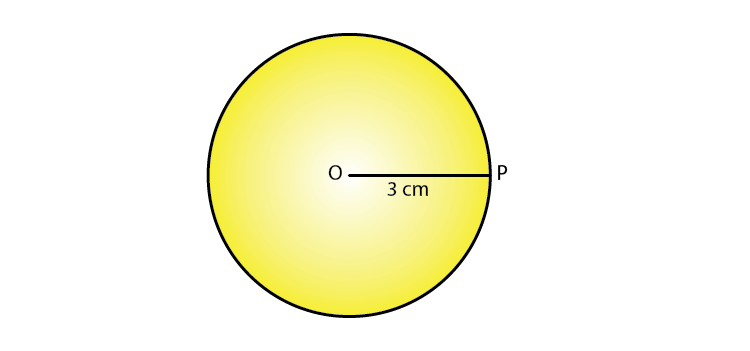The locus of a point which is at a distance of 3 cm away from a fixed point is circumference of a circle whose radius is 3 cm and the fixed point is the centre of the circle.

2. The locus of a points at a distance of 2 cm from a fixed line.

Solution: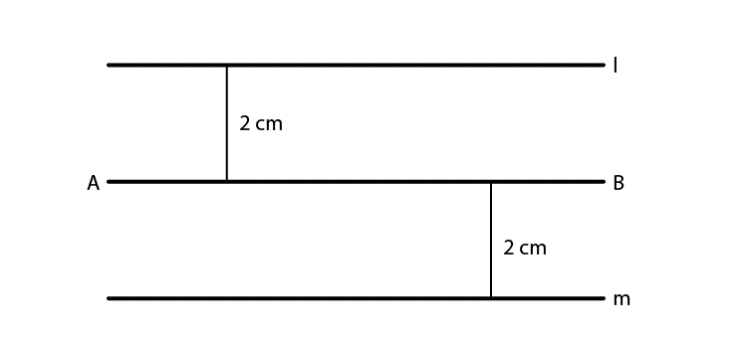The locus of points which are at a distance of 2 cm from a fixed line AB are a pair of straight lines l and m which are parallel to the given line at a distance of 2 cm.

3. The locus of the centre of a wheel of a bicycle going straight along a level road.

Solution: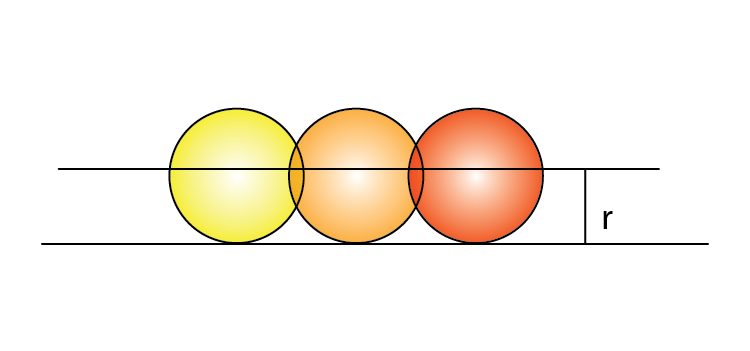The locus of the centre of a wheel, which is going straight along a level road will be a straight line parallel to the road at a distance equal to the radius of the wheel.

4. The locus of the moving end of the minute hand of a clock.

Solution: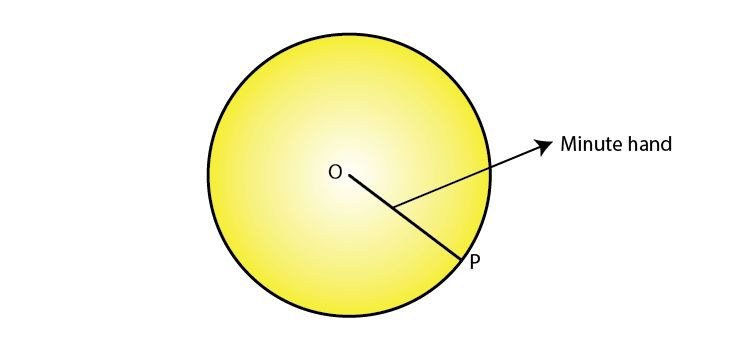The locus of the moving end of the minute hand of the clock will be a circle whose radius will be the length of the minute hand.

5. The locus of a stone dropped from the top of a tower.

Solution: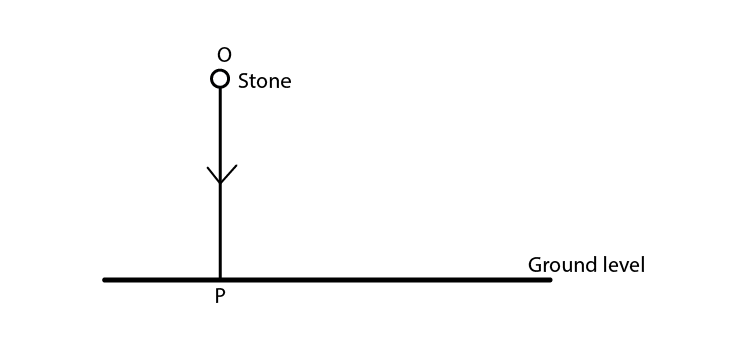The locus of a stone which is dropped from the top of a tower will be a vertical line through the point from which the stone is dropped.

6. The locus of a runner, running around a circular track and always keeping a distance of 1.5 m from the inner edge.

Solution: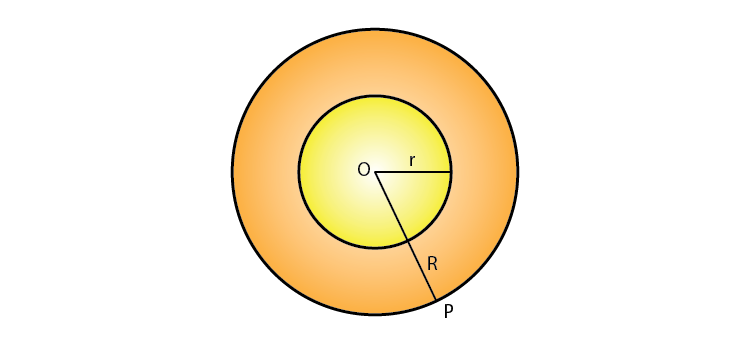The locus of the runner, running around a circular track and always keeping a distance of 1.5 m from the inner edge will be the circumference of a circle where the radius is equal to the radius of the inner circular track and exceeding 1.5 m.

7. The locus of the door-handle, as the door opens.

Solution: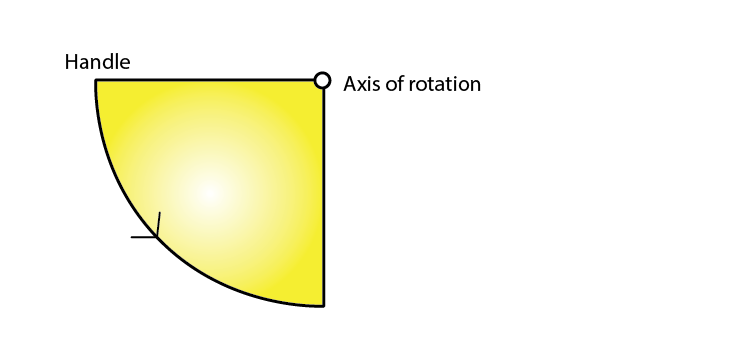The locus of the door handle will be the circumference of a circle with centre at the axis of rotation of the door and the radius equal to distance between the door handle and the axis of rotation of the door.

8. The locus of a points inside a circle and equidistant from two fixed points on the circumference of the circle.

Solution: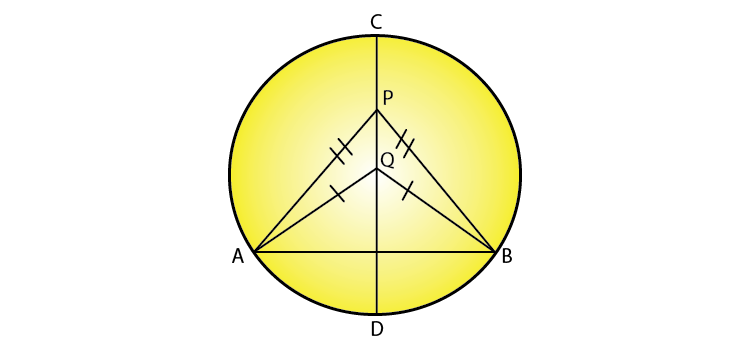The locus of the points inside the circle which are equidistant from the fixed points on the circumference of a circle will be a diameter which is the perpendicular bisector of the line joining the two fixed points on the circle.

9. The locus of the centres of all circles passing through two fixed points.

Solution: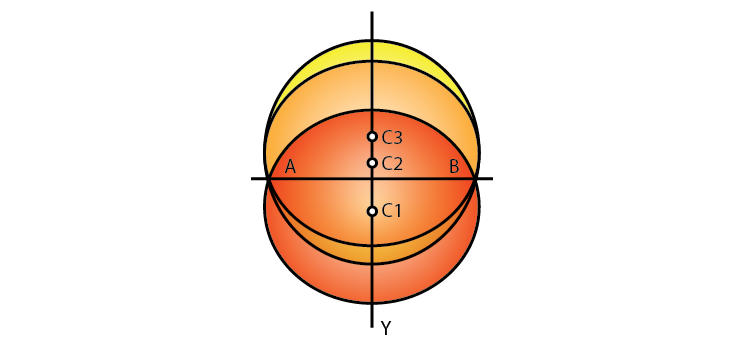The locus of the centres of all the circles passing through two fixed points will be the perpendicular bisector of the line segment joining the two given fixed points.

10. The locus of vertices of all isosceles triangles having a common base.

Solution: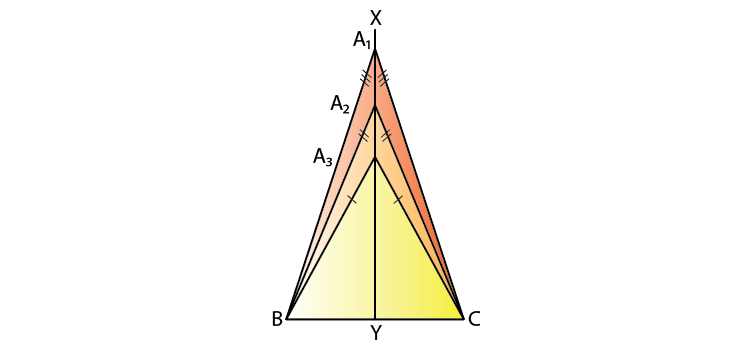The locus of vertices of all isosceles triangles having a common base will be the perpendicular bisector of the common base of the triangles.

11. The locus of a point in space which is always at a distance of 4 cm from a fixed point.

Solution:

The locus of a point in space is the surface of the sphere whose centre is the fixed point and radius equal to 4 cm.

12. The locus of a point P, so that:

AB2 = AP2 + BP2,

where A and B are two fixed points.

Solution: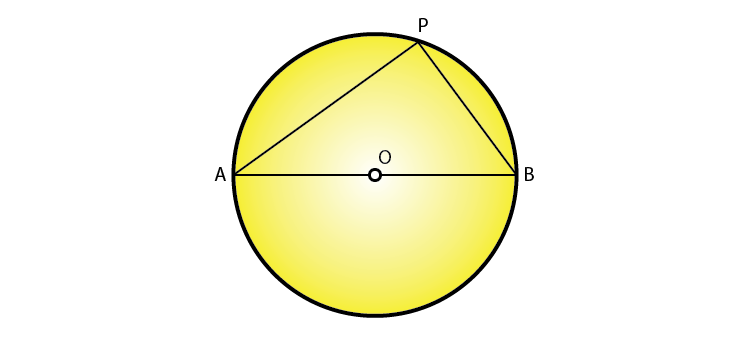The locus of the point P is the circumference of a circle with AB as diameter and satisfies the condition AB2 = AP2 + BP2.

13. The locus of a point in rhombus ABCD, so that it is equidistant from

i) AB and BC; ii) B and D.

Solution:

i)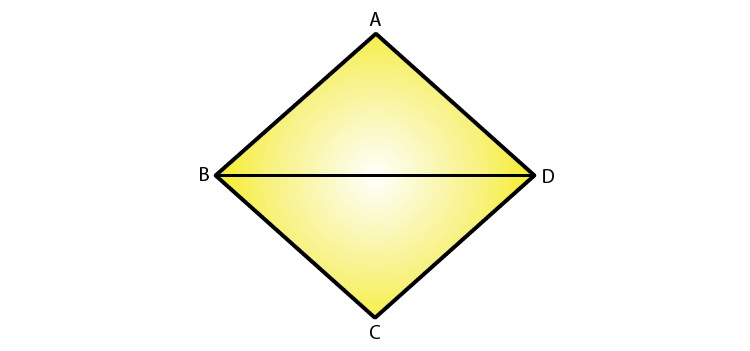The locus of the point in a rhombus ABCD which is equidistant from AB and BC will be the diagonal BD of the rhombus.

ii)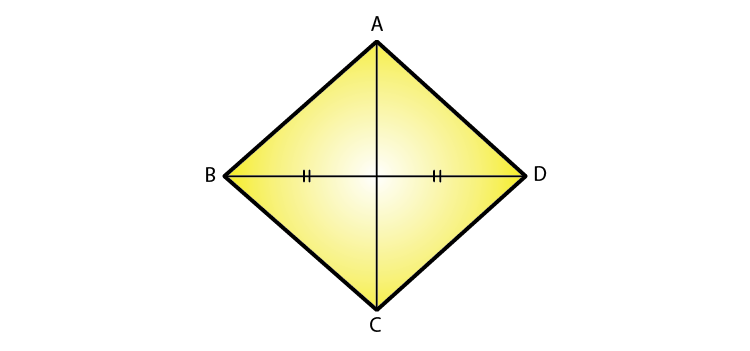The locus of the point in a rhombus ABCD which is equidistant from B and D will be the diagonal AC.

14. The speed of sound is 332 meters per second. A gun is fired. Describe the locus of all the people on the Earth’s surface, who hear the sound exactly one second later.

Solution:

The locus of all the people on Earth’s surface is the circumference of a circle whose radius is 332 m and centre is the point where the gun is fired.

15. Describe:

i) The locus of points at distances less than 3 cm from a given point.

ii) The locus of points at distances greater than 4 cm from a given point.

iii) The locus of points at distances less than or equal to 2.5 cm from a given point.

iv) The locus of points at distances greater than or equal to 35 mm from a given point.

v) The locus of the centre of a given circle which rolls around the outside of a second circle and is always touching it.

vi) The locus of the centres of all circles that are tangent to both the arms of a given angle.

vii) The locus of the mid-points of all chords parallel to a given chord of a circle.

viii) The locus of points within a circle that are equidistant from the end points of a given chord.

Solution:

i) The locus of the points will be the space inside of the circle whose radius is 3 cm and centre as the given point.

ii) The locus of the points will be the space outside of the circle whose radius is 4 cm and centre is the given fixed point.

iii) The locus of points is the space inside and the circumference of the circle with a radius of 2.5 cm and the centre as the given fixed point.

iv) The locus is the space outside and circumference of the circle with a radius of 35 mm and the centre as the given fixed point.

v) The locus is the circumference of the circle concentric with the second circle whose radius is equal to the sum of the radii of the given two circles.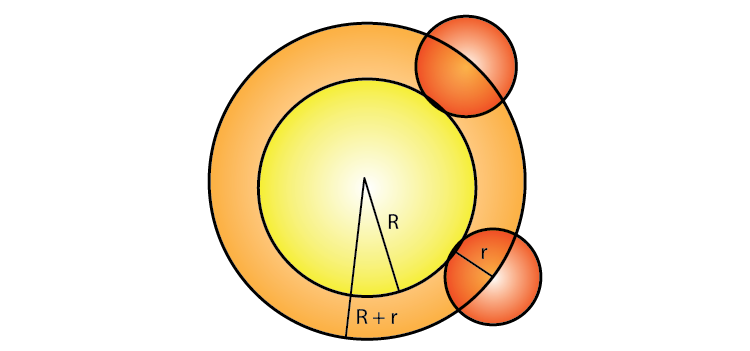vi) The locus of the centre of all circles whose tangents are the arms of a given angle is the bisector of that angle.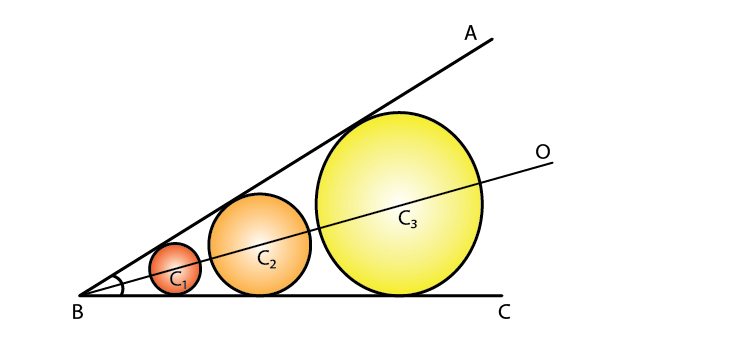vii) The locus of the mid-points of the chords which are parallel to a given chords is the diameter perpendicular to the given chords.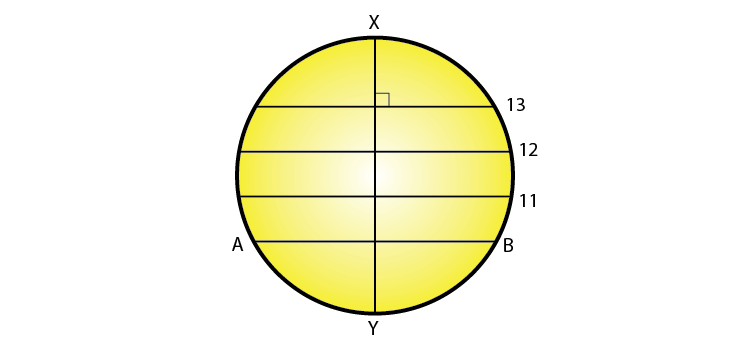viii) The locus of the points within a circle which are equidistant from the end points of a given chord is the diameter which is the perpendicular bisector to the given chord.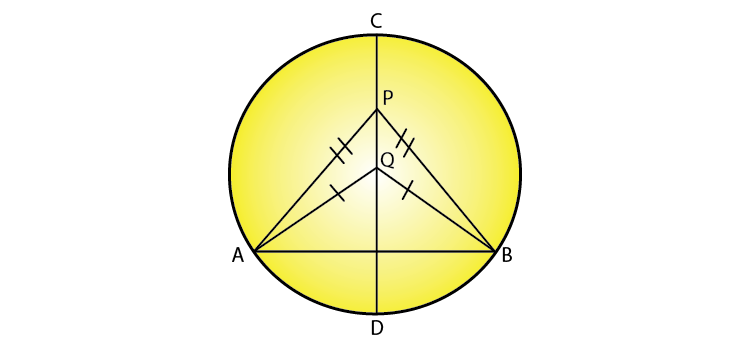### Access another exercise of Selina Solutions Concise Maths Class 10 Chapter 16 Loci (Locus and its Constructions)

Exercise 16(A) Solutions